A smooth distribution on a smooth fibre bundlewith Lie structure group(i.e. a smooth field of linear subspaces of the tangent spaces to) that defines a connection onin the sense that the horizontal liftings of curves in the base manifold are integral curves of this distribution. A horizontal distributionis transversal to the fibres, i.e. at any pointa direct decomposition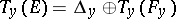holds, where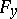is the fibre containing. The additional conditions that must be imposed on a transversal distribution, sufficient to make it a horizontal distribution in the general case, are quite complex. In the particular case ofbeing the total spaceof a principal fibre bundle, they must guarantee the invariance of the distribution with respect to the action of the groupon. In this case these conditions are formulated using the connection forms that have as annihilator the horizontal distribution, and are expressed in the Cartan–Laptev theorem. It follows from the relevant structure equations that if the smooth vector fieldsandonare such that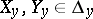at any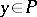, thenhas the component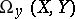in, whereis the curvature form. Thus, a horizontal distribution is involutory if and only if the connection ondefined by it is flat.
A horizontal distribution on a bundleassociated tois always the image of some horizontal distributiononunder canonical projections of the factorizations that are used to constructstarting from. In the general case,is obtained by factorization fromwith respect to the action ofaccording to the formula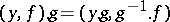. Let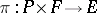be the corresponding canonical projection. Each horizontal distribution onis obtained as the image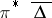, where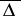is the natural lifting offromto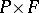. In the more special case whenis a homogeneous space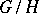, the spaceis identified with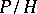and each horizontal distribution onis obtained as the image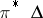under the canonical projection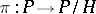.